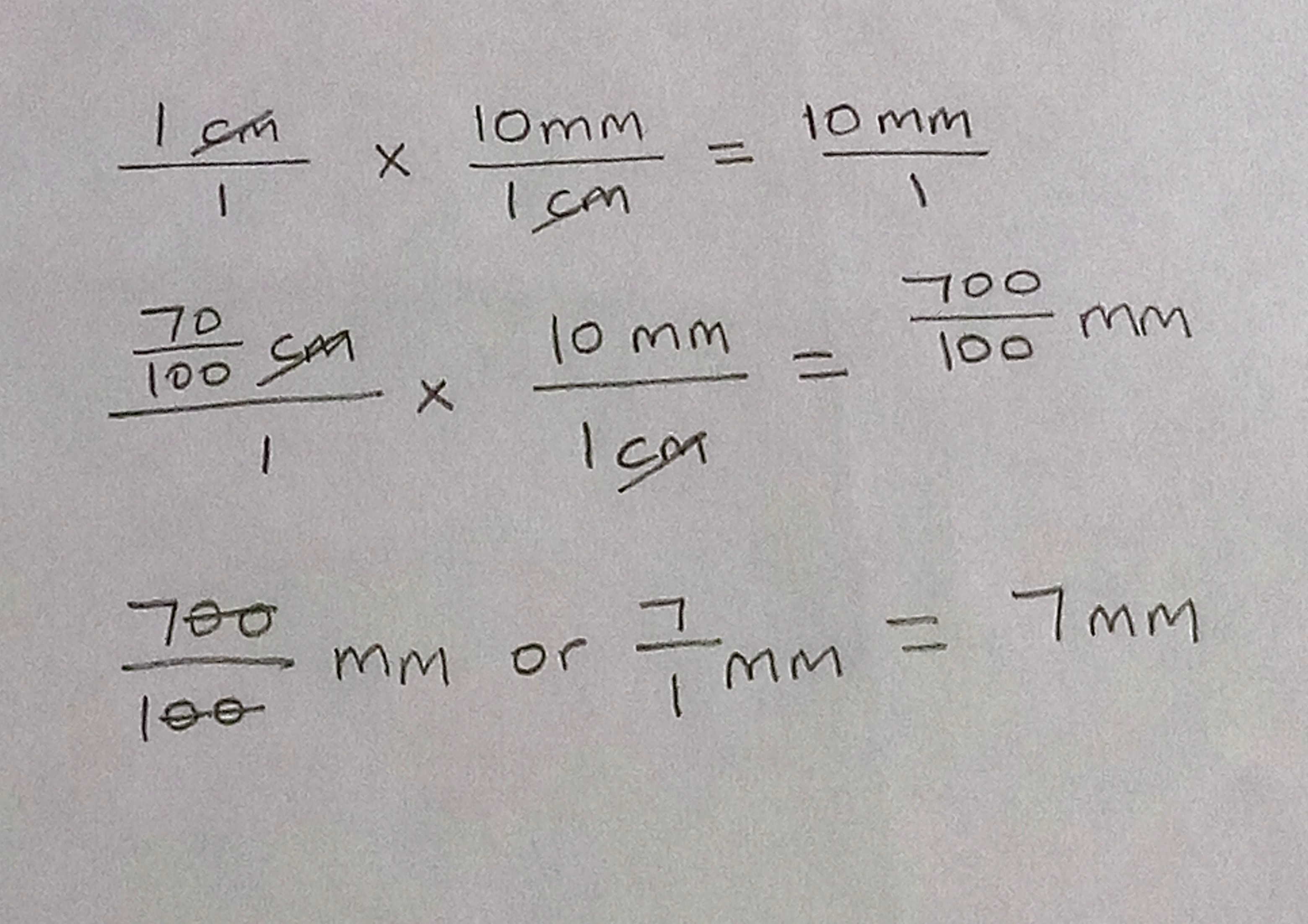Как да помогнем?

### 1 коментар

• Hi jay!

70/100 cm is equal to 7mm. Here's what you can do:

When converting a large unit (cm) to a small unit (mm), multiply the larger unit by the conversion factor.

So, 70/100 x 10 = 700/100

700/100 = 7/1= 7

(I'm going to a a bit complicated here- well it depends :-D) In the image below, I used dimensional analysis, which is like cross multiplication of conversion factors.

Best of luck; have a great week!Постингът е затворена за коментари.